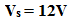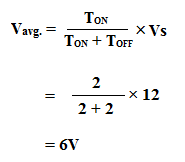## Electronic CHOPPER

Today we are going to discuss Electronic Chopper. In Power Electronics the Chopper is very important Topic. We will discuss how a Chopper works, and its components etc.

If I ask you how can we Step Up or Step Down the AC voltage, you will say using a Transformer, but DC Voltage?
We all know that the Transformer cannot Step Up or Step Down the DC Voltage, that is why we use Electronic Chopper Circuit.

### The Concept of Step Down of DC Voltage:

Suppose we have a DC voltage source of 12V. Now if we want to run a 6V load then we need to reduce the 12V DC to 6V DC.
There is a very easy method of Step down the DC voltage which is by adding a Resistor in series, which will step down the voltage by creating a voltage drop. But we know that if we step down the voltage using the resistor there will be a huge power loss. So this method is not preferable.

Now let's see how we can step down the DC voltage using Chopper circuit,

See all below diagrams carefully to understand easily,

As you see in the above diagram, there is a voltage source of 12V and a load which can operate at 6V. A switch is connected in series between them. Now if we keep ON the switch for 2sec and keep OFF for 2sec and the process remain repeating then the output voltage or voltage across the load will be 6V.
You may think that how it is possible? See the below diagrams you will understand,

The supply voltage,ON time of the switch,OFF time of the switch,So the average voltage or output voltage will be,So you may understand by turning the switch ON, and OFF repeatedly we can step down the DC voltage. Here no power loss occurs. The chopper circuit does the same. So the concept of the Chopper circuit is very simple. Here, the duty cycle is the primary concept for voltage step-down.

### Step Down Chopper:

The Chopper circuit which steps down the voltage is called Step-Down Chopper. It is also called a Buck Converter. A semiconductor switch like SCR(Silicon Controlled Rectifier) is connected between the Source and load. When we give the supply to the gate terminal of the SCR, then it closes the circuit and current will flow from the supply to the load. The pulse signal is given to the gate of the SCR for the switching operation. Basically, the pulse width modulation technique is used to trigger the gate of the SCR.

### Step Up Chopper:

The Chopper which steps up the voltage is called Step-Up Chopper. It is also called a Boost Converter.
In this circuit the ON, OFF process works like a step-down chopper circuit but here is a little different.

You can see in the above figure the switch or SCR is connected in parallel with the load but in case of a step-down chopper, the switch or SCR was connected in series with the load. Here an Inductor is connected in series. When we turn on the switch or SCR then the current will flow through the Inductor and the SCR. The current also flows from the supply to the load.

So during the ON condition of the switch, the voltage across the load will be Zero and the voltage across the inductor is the same as the supply voltage. Suppose the supply voltage is 6V so the voltage across the inductor will be 6V. During ON condition of the switch, the Inductor storing the electrical energy in form magnetic field. Suppose the magnitude of the stored energy in the inductor is 6V.

Now when we turn OFF the switch the stored energy of the Inductor comes in series with the supply voltage and the total output voltage will be (6+6)V = 12V which will be applied across the load.
So, in this case, the average value or output voltage will be greater than the supply voltage.

So the concept of Electronic Chopper is very simple. Just by the ON, OFF operation, an electronic Chopper steps up and step down the DC voltage.

Thank you for visiting the website. keep visiting for more updates.

How does Electronic CHOPPER Step Up and Step Down the DC Voltage?Reviewed by manoranjan das on 10:08 am Rating: 5